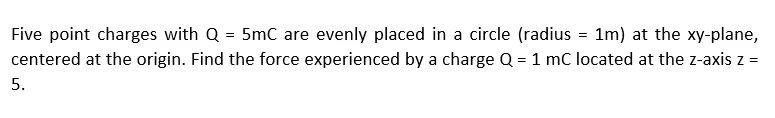# Question Solved1 AnswerFive point charges with Q = 5mC are evenly placed in a circle (radius = 1m) at the xy-plane, centered at the origin. Find the force experienced by a charge Q = 1 mC located at the z-axis z = 5. Five point charges with $$Q=5 \mathrm{mC}$$ are evenly placed in a circle (radius $$=1 \mathrm{~m}$$ ) at the $$x$$-plane, centered at the origin. Find the force experienced by a charge $$Q=1 \mathrm{mC}$$ located at the $$z$$-axis $$z=$$ 5.UG5BZR The Asker · Electrical Engineering

Five point charges with Q = 5mC are evenly placed in a circle (radius = 1m) at the xy-plane, centered at the origin. Find the force experienced by a charge Q = 1 mC located at the z-axis z = 5.Transcribed Image Text: Five point charges with $$Q=5 \mathrm{mC}$$ are evenly placed in a circle (radius $$=1 \mathrm{~m}$$ ) at the $$x$$-plane, centered at the origin. Find the force experienced by a charge $$Q=1 \mathrm{mC}$$ located at the $$z$$-axis $$z=$$ 5.
More
Transcribed Image Text: Five point charges with $$Q=5 \mathrm{mC}$$ are evenly placed in a circle (radius $$=1 \mathrm{~m}$$ ) at the $$x$$-plane, centered at the origin. Find the force experienced by a charge $$Q=1 \mathrm{mC}$$ located at the $$z$$-axis $$z=$$ 5.